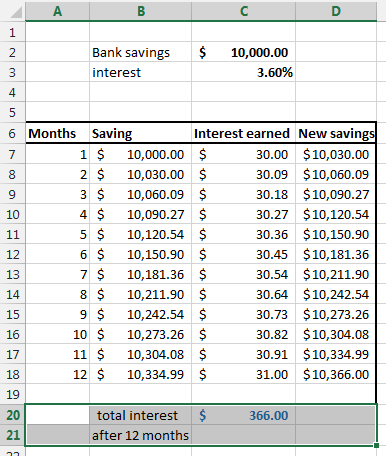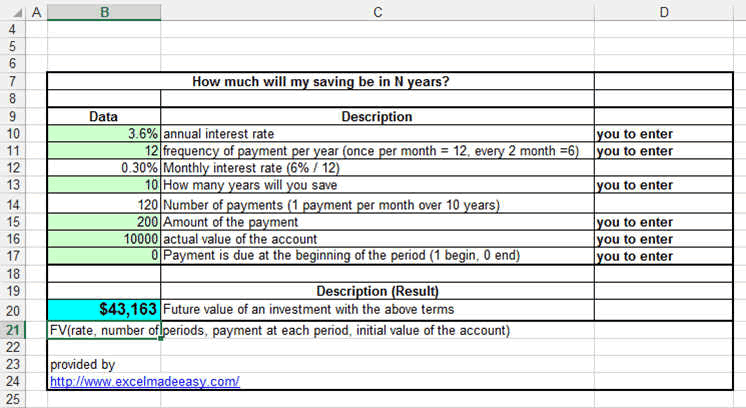## Bank Interest in Excel

You have a loan at the bank, and you want to know how much interest you have to pay per month or year.... here how:

So you have saved money on your bank account and the bank rewards you with an interest of 3.6% per year.

How much do ist make over 10 years you ask yourself.

Interest is calculated on a monthly base so the 10 years are actually 10 x 12 = 120months

Every month, the amount you have is increased by the monthly interest (which is 3.6% / 12 = 0.3%)..... it seems small but small things make great things over time....

So after one month you have \$10'000x0.3% = \$30 more on your account

second month (if you did not withdraw), you will have \$10'030 x 0.3% = 10'060.09

etc....

In excel it looks like:### FV function (future value)

Now we can make it simpler by using the FV (for future value) function that Excel provides us.

=FV(rate per period, number of periods, payment at each period, initial value of the account)

Here is an example how to calculate the money you will have after a certain time.